### IMO Shortlist 2008 problem G4

Kvaliteta:
Avg: 3,0
Težina:
Avg: 7,0
In an acute triangle$ABC$ segments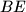$BE$ and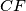$CF$ are altitudes. Two circles passing through the point$A$ anf$F$ and tangent to the line$BC$ at the points$P$ and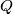$Q$ so that$B$ lies between$C$ and$Q$. Prove that lines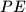$PE$ and$QF$ intersect on the circumcircle of triangle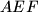$AEF$.

Proposed by Davood Vakili, Iran
Izvor: Međunarodna matematička olimpijada, shortlist 2008